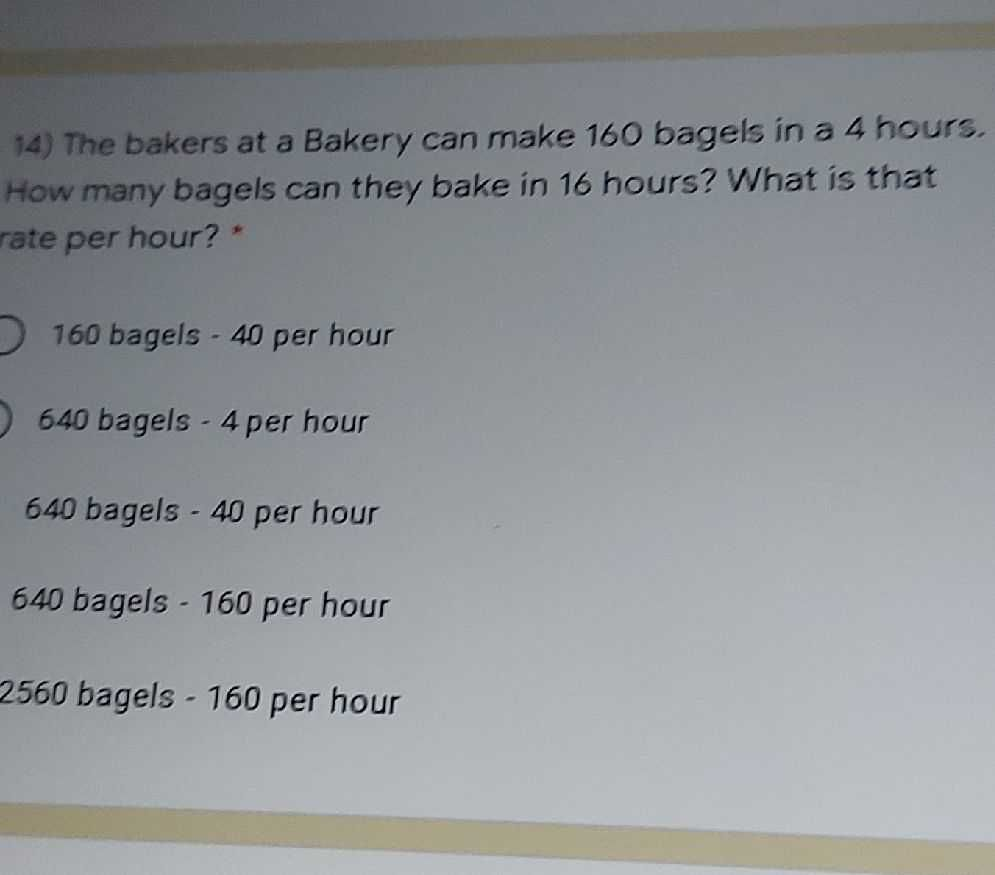### ¿Todavía tienes preguntas de matemáticas?

Pregunte a nuestros tutores expertos
Algebra
Pregunta14) The bakers at a Bakery can make $$160$$ bagels in a $$4$$ hours. How many bagels can they bake in $$16$$ hours? What is that rate per hour? *

$$640$$ bagels - $$4$$ per hour

$$640$$ bagels - $$40$$ per hour

$$640$$ bagels - $$160$$ per hour

$$2560$$ bagels - $$160$$ per hour